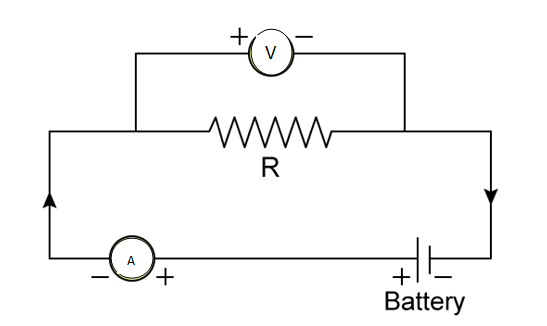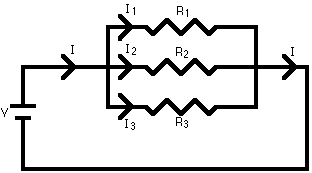# ELECTRICITY#### INTRODUCTION

• Electricity has an important place in modern society.
• The four basic pillars of electricity are voltage, resistance, current and ohm’s law.
• The 4 units of electricity are volts, amps, ohms, and watts.
• The most fundamental law of electricity is ohm’s law.

#### Example:

Let us consider electricity as water flowing in a hose.

The pressure of the water can be said as – Voltage(V)

Size of the hose can be said as – Ampere(A)

Impurities stuck in the hose, which distracts the flow of water can be said as – Resistance### ELECTRIC  CHARGE

Definition:

A charge is a fundamental property of subatomic particles, which experiences a force when placed in an electric field or a magnetic.

• The charge is represented by the symbol (Q)
• Electric charge is a scalar quantity.
• SI unit of Electric charge in Columb.
• Mathematically, the definition of a coloumb is represented as [Q = I.t].

Types:

• Every atom consists of subatomic particles proton, neutron and electron.
•  Proton: It consists of Positive(+) charges.
• Electron: It consists of  Negative (-) charges.
• Neutron: It haves zero charges.

### ELECTRIC CURRENT

• The flow of electrons in an electric circuit with respect to time is called an Electric Current.
• The flow of electrons will be experienced when there is a potential difference in the circuit.
• I = Q/T , I=current ,Q=charge, T=time.
• The current is represented by the letter I.
• The SI unit of current is Ampere.
• Electric Current is measured by a device called an ammeter.

Formula:

I=v/r

where,

I – current,

V – voltage,

R- resistance

### ELECTRIC POTENTIAL DIFFERENCE

• The electron can only flow when there is a difference in electric pressure.
• For example, lets us take the water flowing through a tube. It is only possible when there is high
pressure at one side and low pressure at another side, then it will move from high pressure to low pressure.
• In the case of electric current, the flow of charge is made possible due to chemical action within a cell that generates the potential difference across the two terminals of the cell.

DEFINITION:

It is defined as the work done in carrying a unit charge from one point to another between the two points of an electric circuit.

• SI Unit – Volt (V)
• Voltmeter– A device used to measure the potential difference across two terminals. In other words, to measure the voltage of a circuit.

#### FORMULA:

V=W/Q

V – Potential Difference
W – Work Done
Q – Net Charge

### SYMBOLS FOR CIRCUIT DIAGRAM### OHM’S LAWThe law states that the electric current flowing through a conductor is directly proportional to the potential difference across its terminal.

V ∝ I

V = IR

• R is a constant called resistance for a given metallic wire at a given temperature.
• SI unit of resistance “ohm”.
• The symbol used to represent is Ω.
• 1 Ohm – The resistance of a conductor is said to be 1 Ohm when the potential difference across the conductor is 1V and the current flowing through the circuit is 1A.

### RESISTANCE, RESISTIVITY, RESISTOR

• Resistance– It is the property of a conducting material that opposes or reduces the flow of current. Resistance is represented by ‘R’.
• Resistivity- the resistance offered by a wire of unit length and unit cross-sectional area is called resistivity.
• Resistor –  a device used to reduce the current flow in a circuit.

### RESISTORS IN SERIES CONNECTION

Maximum Effective Resistance

Let us take three resistance R1, R2 and R3 that are connected in series in a circuit.Ohm’s law states,

V = IR

The current (I) flowing through the resistance in series will remain the same, whereas the potential difference (V) across each resistor will differ.Hence, we conclude that the effective Resistance of the several resistors joined in series is equal to the sum of their individual resistances.

#### The disadvantage of series connection in on electric circuit:–

• In a series connection, if any of the components fail to work, the circuit will break and then none of the components (ex. TV, bulb, fan..) will work.
• It is not possible to connect a bulb and a heater in series, because they need different values of current to operate properly.

### RESISTORS IN PARALLEL CONNECTION

Minimum Effective Resistance

Let us take three resistors R1, R2 and R3, that are connected in parallel in the electric circuit.Hence, we conclude that the reciprocal of the total effective resistance of the several resistors connected in parallel is equal to the sum of the reciprocals of the individual resistances.

To overcome this problem with the series connection we generally use the parallel circuits in day to day usage.

### ELECTRIC POWER

In the case of electricity, it is defined as the rate of change in electrical energy consumed in an electric circuit.

P = VI

SI unit of electric power is “Watt” (W).

1 Watt- Defined as the power consumed by an appliance, when 1A of current passes through it at the potential difference of 1V.

P = VI

1 Watt = 1 Volt *1 Ampere